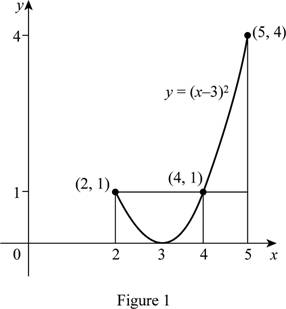# The average value of the function f ( x ) = ( x − 3 ) 2 .### Single Variable Calculus: Concepts...

4th Edition
James Stewart
Publisher: Cengage Learning
ISBN: 9781337687805### Single Variable Calculus: Concepts...

4th Edition
James Stewart
Publisher: Cengage Learning
ISBN: 9781337687805

#### Solutions

Chapter 6.5, Problem 7E

(a)

To determine

## To find: The average value of the function f(x)=(x−3)2.

Expert Solution

The average value of the function is 1.

### Explanation of Solution

Given information:

The function is f(x)=(x3)2,[2,5].

Calculation:

Show the function as below:

f(x)=(x3)2 (1)

The expression to find the average value of the function (favg) is shown below:

favg=1baabf(x)dx (2)

Here, the upper limit is b, the lower limit is a, and the function is f(x).

Substitute 2 for a, 5 for b, and (x3)2 for f(x) in Equation (2).

fave=15225(x3)2dx=1325(x3)2dx (3)

Integrate Equation (3) with respect to x and apply the limits.

fave=1325(x26x+9)dx=13[x336x22+9x]25=13{[533(3×52)+(9×5)][233(3×22)+(9×2)]}=13(3)

=1

Thus, the average value of the function is 1.

(b)

To determine

### To find: The value of count c.

Expert Solution

The value of c is 2 or 4.

### Explanation of Solution

Given information:

The function is f(x)=(x3)2,[2,5].

Calculation:

Find the value of c using the formula:

f(c)=fave (4)

Substitute 1 for fave in Equation (4).

f(c)=1 (5)

Substitute c for x in Equation (1)

f(c)=(c3)2 (6)

Substitute 1 for f(c) in Equation (6).

(c3)2=1c3=1c3=±1 (7)

Find c value using Equation (7).

c3=1c=4

Find the another value of c.

c3=1c=1+3

c=2

Thus, the value of c is 2 or 4.

(c)

To determine

Expert Solution

### Explanation of Solution

Substitute 2 for x in Equation (1)

y=(23)2=1

Substitute 4 for x in Equation (1)

y=(43)2=1

Draw the graph for the curve function y=(x3)2 and mark the coordinates (2,1), (4,1) as shown in Figure 1.### Have a homework question?

Subscribe to bartleby learn! Ask subject matter experts 30 homework questions each month. Plus, you’ll have access to millions of step-by-step textbook answers!